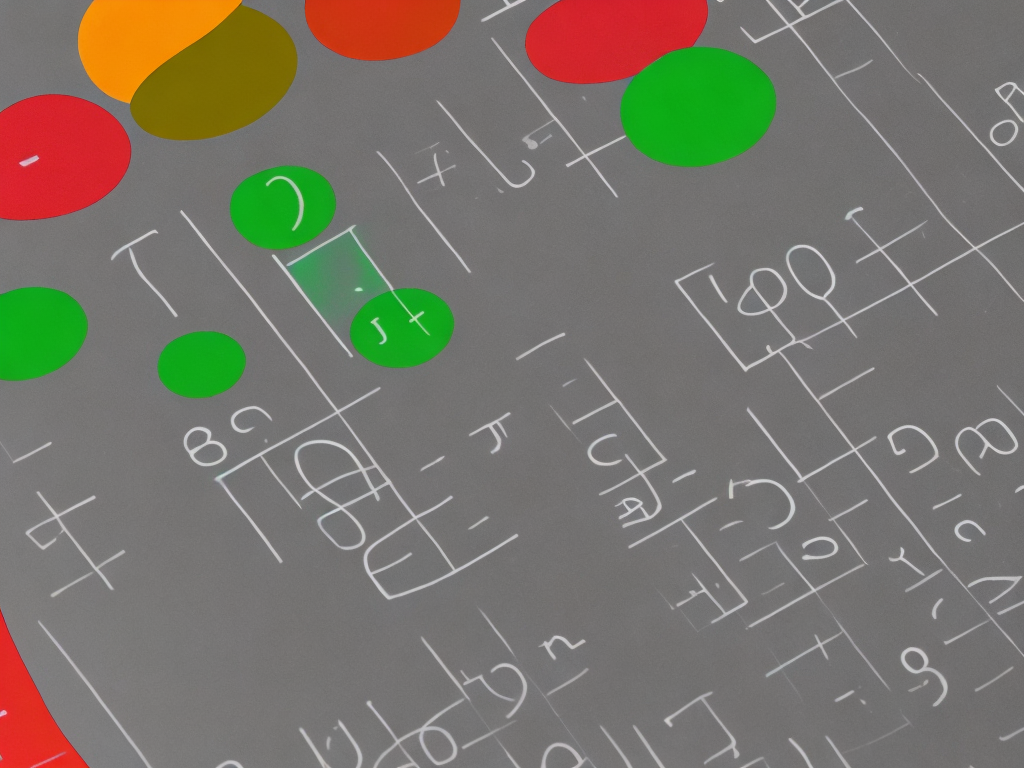# Difference Between Relative And Percentage Errors

tl;dr
The main difference between relative and percentage errors is that relative error is expressed as a decimal metric, while percentage error is expressed in ratio form. Percentage error is a more convenient metric to use as it relates any unit of measurement to a percentage, while relative error is a dimensionless metric, limiting accuracy of comparison to identical measuring units.Errors are an inevitable and inescapable reality when making measurements or conducting experiments. They can arise from a plethora of sources such as inherent machine or instrument limitations, environmental variability, or even human error. Hence, distinguishable types of errors require comprehension to appreciate their significance explicitly in quantification. Two of the most commonly misconceived types of errors are relative and percent errors.

This article explains the difference between relative and percentage errors, their significance in measurement, and how to calculate them.

What is errors?

Errors are the difference between an actual value and the measured or observed value. They arise from various sources, such as inherent machine or instrument limitations, environmental variability, or even human error. In research, errors in measurements or observations can skew the validity and extremity of resulting measurements. It, therefore, becomes paramount to determine and minimize errors in all measurements.

What is Relative Error?

Relative error, also called the fractional error, is the difference between the measured and actual values divided by the actual value. It is a proportion of the amount of error in respect to the true value or the magnitude of the measurement. Relative error is a dimensionless quantity, making it suitable for comparing errors of different magnitudes and scales.

Relative error is important in measuring accuracy in regards to decimal places, where the numerator presents the inaccuracy quantity while the denominator shows the precision of the measurement. Relative error is a straight forward technique for quantifying how close or how far the measurement is from the actual value.

Real-world applications of Relative Error

Relative error is exceptionally convenient in practice, particularly in fields like metrology, physics, and engineering. In electronics, for example, relative error is key in determining the accuracy, precision, and ranges of battery voltages, power transmission, and voltage amplifiers. Additionally, it is used in measuring the accuracy of humidity and temperature sensors. The measurement result of any sensor may be compared to a standard value using relative error, allowing for a closer variation assessment of the statistical characteristic.

Calculating Relative Error

To calculate relative error, the formula below is used:

Relative error = (Measured value - Actual value) / Actual value

For instance, If the actual value of a measurement is 100, and the measured value is 90, then the relative error would be:

Relative error= (90 - 100) / 100 = -0.1

What is Percentage Error?

Percentage error is the ratio of the difference between the measured and actual values divided by the actual value, then multiplied by 100. It is the unit of relative error and expresses the error in percentage form. Like relative error, it helps in quantifying the accuracy of a measurement.

In essence, percentage error is a relative error metric shown in percentage rather than a decimal. It is used primarily to see how the actual and measured values differ in terms of a specific value.

Real-world applications of Percentage Error

Percentage error is widely utilized in multiple subject areas to determine the accuracy and precision of measurements, particularly in laboratories and research centers. Geographically, the percentage error is used to assess the scale and potential consequences of error in meteorological predictions and geological measurements. Researchers, particularly in fields that require precise measurements, use percentage error to evaluate the accuracy and precision of their experiments.

Calculating Percentage Error

To calculate for percentage error apply the coefficient of 100 to the relative error formula, such that:

Percentage error= Relative error x 100%

Continuing with the previous example, if the actual value of a measurement is 100, and our measured value is 90, then the percentage error would be:

Percentage error= (-0.1) x 100% = -10%

Difference Between Relative and Percentage Errors

The primary difference between relative and percentage errors is the used scales. Relative error is applied to measurements as a decimal metric, while percentage error is expressed in ratio form. For instance, 0.05 is the relative error, whereas 5% is the percentage error.

Consequently, percentage error is a more convenient metric to use given that it relates any unit of measurement to a percentage, making it easier to compare measurements across subjects. Relative error, on the other hand, is a dimensionless metric, limiting the accuracy of comparison to identical measuring units.

Conclusion

Errors are inevitable in any research, observation, or experiment, hence distinguishing between the different types of errors is fundamental to improve the accuracy and precision of measurements. Relative and percentage errors are critical metrics used in real-life applications of various fields such as physics, engineering, meteorology, and others that require precise measurements.

While percentage error is a more convenient metric to use, specialists need to make accurate comparisons across different measurements, irrespective of the measurement unit. Hence, it is paramount to have an accurate understanding of both to correctly assess the scale and potential implications of errors. With both relative and percentage error, response to variable revisions in a measurement or experiment can be easier, and the possibility of error minimized.